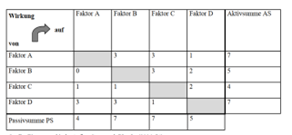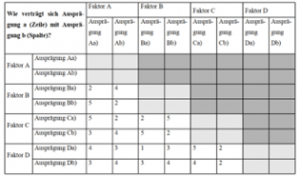## Scenario Planning

Watch
Do

The scenario technique is a method of strategic planning used in politics, science and business. The aim is to analyse possible developments of the future and to present them in a coherent way. The understanding of the future underlying the scenario technique is characterised above all by the fact that it is not assumed that there is one necessary future, but rather several possible futures. The concept “scenario” stands for the idea of a possible future and thus implicitly always refers to the possibility of further, alternative futures.

The development of a scenario usually consists of five different phases:

Phase 1: Scenario field definition

• For which subject should the scenario be developed?
• What is the subject and the problem to be addressed?
• Where are the boundaries? What is not considered?

Phase 2: Identification of key factors

• Key factors or “descriptors” are central variables that describe the scenario field.
• Key factors = variables, parameters, trends, developments and events
• Definition of key factors:
• empirical preliminary work (often desk research)
• participative in workshops or interviews

Phase 3: Analysis of the key factors

• Analysis of key factors according to possible future characteristics
• A separate future funnel is opened for each factor

Phase 4: Scenario generation

• Scenarios are identified and condensed from the various scenario funnels in the cross-section
• Consistent factor bundles are compiled and selected

Phase 5: Scenario transfer

• Use and / or processing of the scenario
• Various options ( impact analysis, stakeholder analysis, strategy development and evaluation, etc.)

An impact analysis can be carried out to identify the scenarios. This works as follows:

In a matrix, the list of identified factors is noted in the column and row in the same order. Per factor pair we ask the asked: “To what extent is a direct relationship effective between the factors?”Kosow et al. 2008, S.38

Scale to quantify influence:

0 = no influence; 1 = weak relationship; 2 = medium relationship; 3 = strong relationship.

All combinations are scored, leaving the centre diagonal of the matrix blank.  Then row and column sums are calculated: The row sum = “active sum” (AS), indicates how strongly the factor affects other factors.  The column sum = “passive sum” (PS), indicates how strongly the factor is influenced by other factors. Key factors with a high active sum and those with a high active sum AND a high passive sum are used for further scenario construction.

To ensure the consistency of the scenario, a consistency analysis follows with the selected key factors: Each factor is given two different characteristics. All factor characteristics are compared with all other factor characteristics.For each combination, the consistency is assessed and rated on a scale of 5.Kosow et al. 2008, p.38

5 = strong consistency (strong mutual support); 4 = weak consistency (mutual favouring), 3 = neutral or independent of each other, 2 = weak inconsistency (contradiction)1 = strong inconsistency (total contradiction)

The matching factors are then used to create different scenarios.

• Watch

Scenario technique

• Do

UseCase: Immigration (40 min work)

Short description: It is the year 2035 – How open is Germany to the issue of immigration, refugees and integration? Which scenarios can be outlined here?

Discuss the case and the question. What are the key factors? Select 8 key factors. Use the impact analysis and the consistency analysis to evaluate your key factors.Sketch & name 2-4 rough scenarios that emerge from your analysis. Describe your scenarios. Explain the advantages and disadvantages of your method. What worked well? What information was missing?